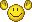# What is your user number?

#### Xexyz

##### GBATemp's™ Official Xexyz
Member
To find out here's how!As you can see I am user 332,593.
If you want to make it easier for me.
(tell me if there is an error)
Here's a template!

#(number here)=(name (color if staff))=(newcomer, member, staff etc)
#1 = KiVan = Former Staff/ Creator#25 = Thug = Reviewer
#158 = fluffykiwi = Member
#746 = Cyan = Global Moderator
#1,476 = Blood Fetish = Member
#4,500 = Zarcon = Reviewer
#18,561 = Blebleman = Member
#24,554 = Youkai = Member
#32,627 = Master Mo = Member
#35,335 = jamesaa = Member
#38,716 = Zharktas = Newcomer
#43,450 = Chanser = Reporter
#60,228 = player594 = Member
#80,085 = RedCoreZero = Member
#89,442 = kaz_abdin = Member
#97,900 = Minox = Former Staff
#103,388 = dragonblood9999 = Member
#109,594 = BortzANATOR = Member/ (bortz guy)
#114,997 = Ryukouki = Member
#123,159 = p1ngpong = Supervisor
#124,912 = Lacius = Member
#128,123 = porkiewpyne = Member
#130,867 = SignZ = IRC Staff
#132,061 = Langin = Member
#138,644 = Depravo = Global Moderator, Adventurer, Sharpshooter, Bareback Horseman, Sailor of the Seven Seas, Lord of the Dance and Best Dressed Man of 1982.
#139,899 = air2004 = Member
#141,797 = Ericthegreat = Member
#143,466 = Blaze163 = Member
#152,102 = tj_cool = Supervisor
#155,560 = emigre = Member
#157,113 = AaronUzumaki = Member
#161,107 = ThatDudeWithTheFood = Member
#172,712 = Hells Malice = Member
#183,192 = GameWinner = Member
#187,316 = Tom Bombadildo = Member
#187,870 = Sicklyboy = Member
#188,859 = Thomas83Lin = Member
#193,826 = Devin = Reviewer
#197,789 = DarkShinigami = Member
#200,556 = _Chaz_ = Member
#202,697 = The Catboy = Member
#210,386 = moerik = Newcomer
#233,985 = Tonitonichopchop = Member
#244,492 = Rydian = Content Manager
#244,868 =NahuelDS = Member
#250,252 = gameplayer_9198 = Newcomer
#251,087 = anthonyplep = Member
#251,644 = Liberta = Member
#258,755 = ilman = Member
#260,705 = nukeboy95 = Member
#262,066 = Arras = Member
#263,182 = Ethevion = Member
#264,630 = Attila13 = Member
#267,288 = Sop = Member
#268,560 = Yepi69 = Member
#269,655 = BrightNeko = Member
#272,236 = marksteele = Member
#275,395 = linuxGuru = Member
#280,473 = riyaz = Member
#282,323 = Gahars = Member
#282,761 = Par39 = Newcomer
#284,109 = ComeTurismO = Member
#284,338 = pyromaniac123 = Member
#285,041 = DinohScene = Member/Temp's Official femboy/ 360 hacker
#286,420 = Black-Ice = Member
#286,466 = GamerzHell9137 = Member
#288,500 = beta4attack = Member
#291,886 = Nxenz = Member
#318,366 = dj4uk6cjm = Member
#319,129 = Isaac = Member
#330,881 = Vengenceoncu = Member
#322,791 = mr allen = Newcomer
#329,878 = calmwaters = Member
#329,988 = drottning = Member/silly catgirl
#332,057 = Duo8 = Member
#332,593 = Xexyz = Member
#333,503 = SmokeyTheDog = Newcomer

Member
330,881

#### DarkShinigami

##### #1 strongest Shinigami BANKAI
Member
197789

dont see the point but contributing

Member
275395

#### nukeboy95

##### Old skool member
Member260,705

#### Sop

##### groovy dude lmao
Banned
-1.279 + 2 - 1.279

#### Xexyz

##### GBATemp's™ Official Xexyz
Member
-1.279 + 2 - 1.279
what does it mean?Member
233,985.

#### porkiewpyne

##### Report-er
Supervisor
128123#### Xexyz

##### GBATemp's™ Official Xexyz
Member
Added all of you in + others,

#### Gameplayer9198

##### Well-Known Member
Member
250252Former Staff
97990

#### beta4attack

##### Well-Known Member
Member
288500 here#### Arras

##### Well-Known Member
Member
Not particularly useful but hey. 262066.

Member
I'm 258755.

#### mr allen

##### Well-Known Member
Newcomer
I don't see the point, but oh well. 322791

#### Black-Ice

##### Founder of the Church of Renamon
Member
286420

•EZ-Megaman

#### Langin

##### HI! ^O^
Member
132061

•EZ-Megaman

Member
282.671

#### emigre

##### i am a free i am not man a number
Member

•NahuelDS

•Replies
47
Views
1K

### Recent Content

12. #### Not sure if this goes here but I'm having a problem with Retroarch..

Help Users
• No one is chatting at the moment.